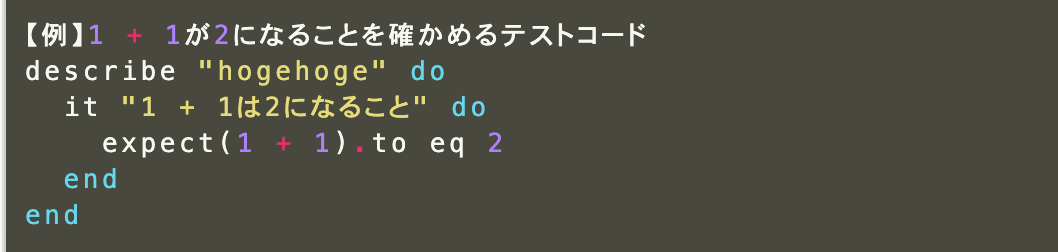# About app testing RSpec (unit test)

RSpec A test-specific language created based on Ruby

# Test code basicsdescribe The describe on the first line creates a group of tests from do to end immediately after. In the "" that follows the describe, write a description of the group.

## it and example

The second line, it, represents a set of working test code called an example. Write a description of the example in the "" that follows it.

## Expectation

It is the formula that is actually evaluated. Write between it do ~ end. In the above equation, the part of expect (1 + 1) .to eq 2 is the expectation.

expect(X).to eq Y Expectation grammar. If the value of the expression in the x part is equal to the value in the Y part, the test succeeds. The part of eq is called matcha.

## Matcha

Shows the conditions under which the test will succeed in the expectation. For example, eq means "if equal". Other There are multiple matchers such as include (if included) and valid (if validated).